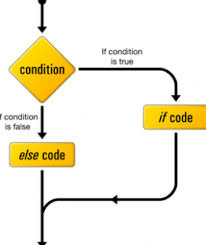Want to share your content on R-bloggers? click here if you have a blog, or here if you don't.Answers to the exercises are available here.

Exercise 1

What is the output of:
`ifelse(sqrt(9)<2,sqrt(9),0)`

Exercise 2

What is the output of:
`ifelse(sqrt(100)>9,sqrt(100),0)`

Exercise 3
What is the output `y` of:
`x=12`
`if(is.numeric(x)) y=x*2`

Exercise 4

What is the output `x,y` of:

`z=-1`
`if(z<0){x=abs(z);y=z*3}`

Exercise 5

What is the output `y` of:
`z=6`
`if(z<0) y=z*3 else y=z*5`

Exercise 6

What is the output `z` of:
`x=15`
`y=3`
```if(is.numeric(x)) if(is.numeric(y) & y!=0) z=x/y```

Exercise 7

What is the output of:
`x=letters`
```if (is.numeric(x)) print('is numeric') else if(is.character(x)) print('is character')```

Exercise 8

What is the output of:
``` x=90 ifelse(x<100,ifelse(x/2==trunc(x/2),x/2,0), ifelse(x/100==trunc(x/100),x/100,-1)) x=120 ifelse(x<100,ifelse(x/2==trunc(x/2),x/2,0), ifelse(x/100==trunc(x/100),x/100,-1)) x=200 ifelse(x<100,ifelse(x/2==trunc(x/2),x/2,0), ifelse(x/100==trunc(x/100),x/100,-1)) ```

Exercise 9

What is the output `n` of:
``` z='i' if (z %in% letters) if (z=='a') n=1 else if (z=='e') n=2 else if (z=='i') n=3 else if (z=='o') n=4 else n=5 ```

Exercise 10

What is the output `n` of:
``` z='u' if (z %in% letters) if (z=='a') n=1 else if (z=='e') n=2 else if (z=='i') n=3 else if (z=='o') n=4 else n=5 ```